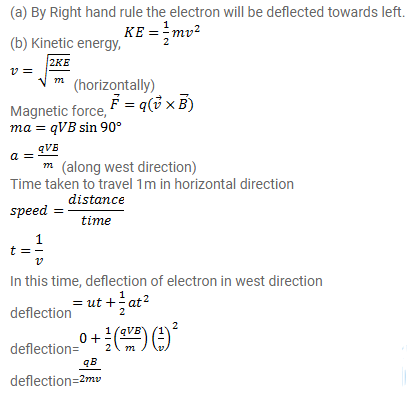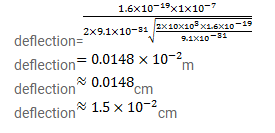# An electron is projected horizontally with a kinetic energy of

Question:

An electron is projected horizontally with a kinetic energy of $10 \mathrm{keV}$. A magnetic field of strength $1.0 \times 10^{-7} \mathrm{~T}$ exists in the vertically upward direction.

(a) Will the electron deflect towards right or towards left of its motion?

(b) Calculate the sideways deflection of the electron in travelling through $1 \mathrm{~m}$. Make appropriate approximations.

Solution: